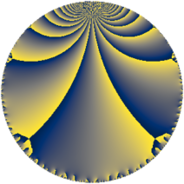# Properties

 Label 441.6.baLevel $441$ Weight $6$ Character orbit 441.ba Rep. character $\chi_{441}(22,\cdot)$ Character field $\Q(\zeta_{21})$ Dimension $3336$ Sturm bound $336$

# Related objects

## Defining parameters

 Level: $$N$$ $$=$$ $$441 = 3^{2} \cdot 7^{2}$$ Weight: $$k$$ $$=$$ $$6$$ Character orbit: $$[\chi]$$ $$=$$ 441.ba (of order $$21$$ and degree $$12$$) Character conductor: $$\operatorname{cond}(\chi)$$ $$=$$ $$441$$ Character field: $$\Q(\zeta_{21})$$ Sturm bound: $$336$$

## Dimensions

The following table gives the dimensions of various subspaces of $$M_{6}(441, [\chi])$$.

Total New Old
Modular forms 3384 3384 0
Cusp forms 3336 3336 0
Eisenstein series 48 48 0

## Trace form

 $$3336 q - 5 q^{2} - 10 q^{3} + 4411 q^{4} - 105 q^{5} + 326 q^{6} - 35 q^{7} - 148 q^{8} - 960 q^{9} + O(q^{10})$$ $$3336 q - 5 q^{2} - 10 q^{3} + 4411 q^{4} - 105 q^{5} + 326 q^{6} - 35 q^{7} - 148 q^{8} - 960 q^{9} - 148 q^{10} - 5 q^{11} + 2165 q^{12} + 357 q^{13} + 2920 q^{14} - 1582 q^{15} + 69691 q^{16} + 5672 q^{17} + 2930 q^{18} - 172 q^{19} - 8837 q^{20} - 9174 q^{21} - 133 q^{22} + 160 q^{23} - 8330 q^{24} + 168745 q^{25} + 29452 q^{26} - 10093 q^{27} + 1576 q^{28} + 29845 q^{29} - 19782 q^{30} + 2156 q^{31} + 5735 q^{32} - 17530 q^{33} + 59 q^{34} - 3404 q^{35} + 136862 q^{36} - 25810 q^{37} - 7513 q^{38} - 28904 q^{39} + 8165 q^{40} + 12571 q^{41} - 112676 q^{42} + 18483 q^{43} - 40720 q^{44} - 93531 q^{45} + 94652 q^{46} + 2945 q^{47} + 11098 q^{48} - 4295 q^{49} - 19554 q^{50} + 9918 q^{51} - 61957 q^{52} + 340496 q^{53} + 52014 q^{54} - 155380 q^{55} - 448745 q^{56} - 62976 q^{57} + 24947 q^{58} - 63859 q^{59} + 142228 q^{60} + 77120 q^{61} - 233964 q^{62} - 123738 q^{63} - 2166932 q^{64} + 23209 q^{65} - 273576 q^{66} - 1258 q^{67} + 727078 q^{68} - 429770 q^{69} + 647 q^{70} + 274802 q^{71} - 29296 q^{72} - 84048 q^{73} + 263907 q^{74} - 405156 q^{75} - 1285 q^{76} + 7891 q^{77} - 405306 q^{78} + 46958 q^{79} - 2233424 q^{80} + 572344 q^{81} - 148 q^{82} - 800291 q^{83} + 303316 q^{84} + 6245 q^{85} - 44793 q^{86} - 738854 q^{87} + 61499 q^{88} + 325858 q^{89} - 9528 q^{90} - 143760 q^{91} + 142289 q^{92} - 745340 q^{93} - 96373 q^{94} - 46358 q^{95} + 1945941 q^{96} - 69370 q^{97} - 2361228 q^{98} - 2070100 q^{99} + O(q^{100})$$

## Decomposition of $$S_{6}^{\mathrm{new}}(441, [\chi])$$ into newform subspaces

The newforms in this space have not yet been added to the LMFDB.